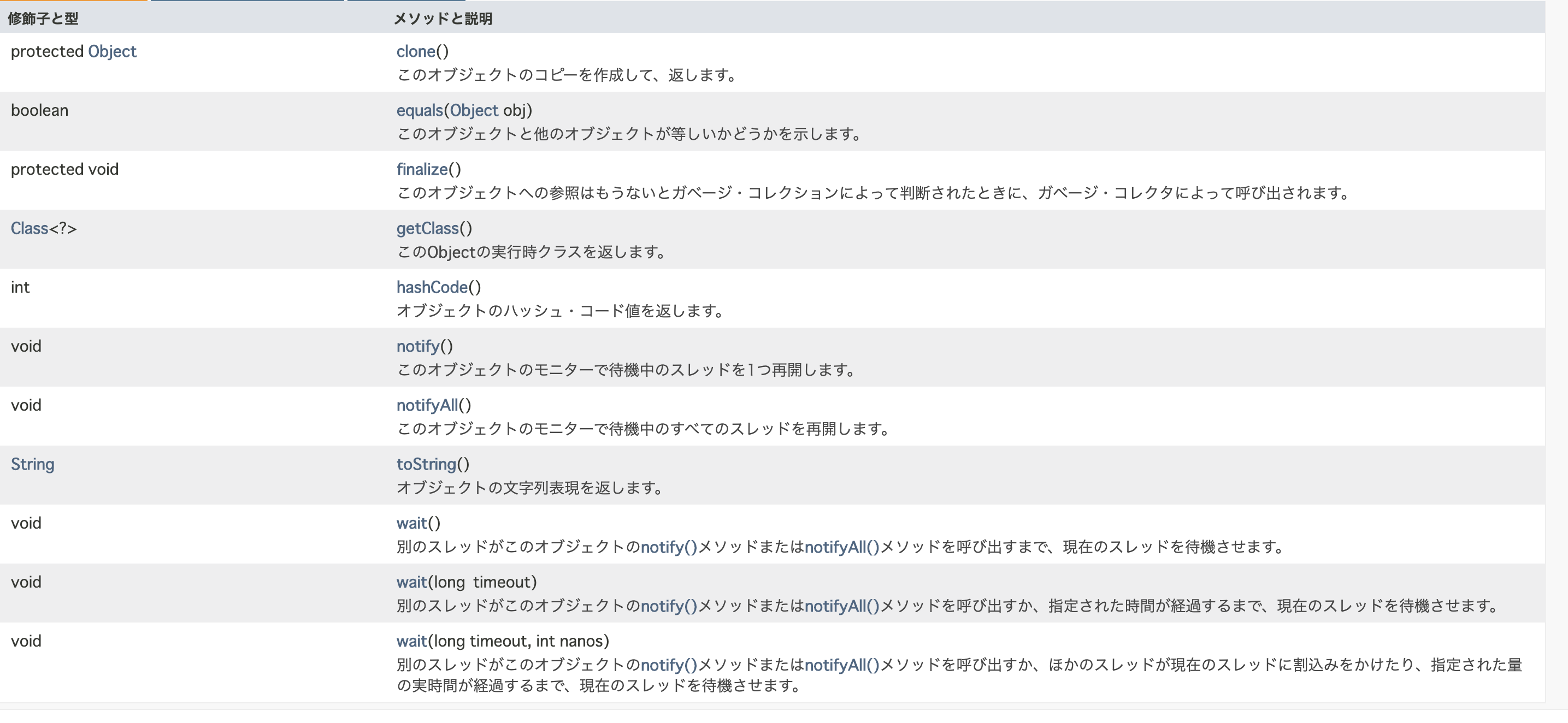# [Java Silver] About equals method

Here's a summary of the equals methods you should remember when studying Java Silver.

## What is the equals method?

equals method

The equals method is a method defined in ** java.lang.Object class **.

## Normal behavior

``````a.equals(b);
``````

Check if the instance stored in variable a and the instance stored in variable b have ** the same value ** ** (equivalence) **

• Use the ** == operator ** to check the ** identity (whether they have the same reference) ** of the instance. Using the ** intern method ** to compare instances that are equivalent but not identical with the == operator returns true
``````String a = "abc";
String b = new String("abc");

System.out.println(a == b); //false is returned
System.out.println(a.intern() == b.intern()); //returns true
``````

This is because the intern method is a method for finding and reusing strings in memory, including constant pools. A constant pool means that when a ** character literal ** reappears in a program, ** references to the same string instance created in the past are reused **.

## Equals method of Object class

Since the equals method is defined in the Object class, every class has it. However, only the equals method of the ** Object class ** is defined to determine ** identity ** rather than equivalence. The definition is as follows.

``````public boolean equals(Object obj){
return (this == obj);
}
``````

Since the ** equivalence confirmation method ** differs for each class, it is assumed that the equals method is ** overridden and used **.

## That it is a method defined in java.lang.Object class

Override the method defined in ** java.lang.Object class ** as ** default method ** in ** interface ** → ** Compile error **

Therefore, when overriding the equals method, it must be overridden in ** class **.

** * List of methods defined in java.lang.Object class **## NullPointerException does not occur even if null is used as an argument

Passing null to the equals method does not result in a NullPointerException

Official document

x.equals (null) returns false.

When a.equals (b), if a is null, a NullPointerException will occur. When a.equals (b), if a is not null and b is null, false is returned. Objects.equals (a, b) added in Java7 does not generate a NullPointerException even if a and b are null.

# reference

Sample comparing null or empty string with Java equals